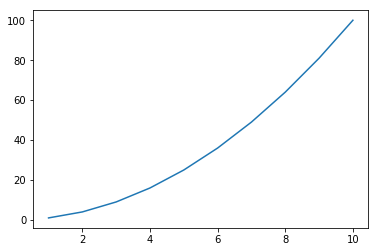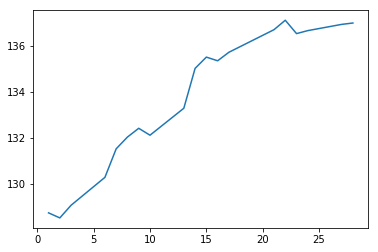Congratulations on getting set up with Python! If you're not familiar with programming then don't worry; you have already done the hardest part. Python is one of the easiest programming languages to get into due to simple syntax (how your code is written), and a highly active community (hello!) - but can be built upon extensively to perform complex data analysis, create websites, and automate typically difficult jobs to be done quickly and efficiently.

In this guide we're going to go over some critical programming concepts to understand, and then move onto creating a simple graph using a library we'll spend a lot of time with - matplotlib. Let's go!

## Programming Basics¶

To start, we need to talk very generally about objects. An object can be generally be thought as a piece of information, such as a number, word, or sentence. Later on we'll see some more complex examples of objects (and eventually how to define our own!) but for now let's look at some simple ones. Since sometimes we want to store an object in our computer's memory to recall later, we can assign an object a label. We call this label a variable.

In :
myVariable = 30


To recall this variable's value, we can use the print function:

In :
print(myVariable)

30


Variables are especially helpful when the object we want to asign them to are very large. Imagine having a dataset of millions of values; it would be very fustrating to write it all out again and again! Another important property of variables is they act just like the object they were assigned to.

In :
apples = 4
oranges = 6

In :
print(apples + oranges)

10


We can assign any object to a variable - since the sum of our two variables above is an object (a number), we can assign it a name.

In :
fruits = apples + oranges

In :
print(fruits)

10


So far, we've only looked at whole numbers being stored as variables. In Python, these are called integers or int. For any variable, we can check the type by using the type() function.

In :
print(type(fruits))

<class 'int'>


Later we'll see how to define our own types, but let's look at some of the more common ones. First off we have floating point numbers.

In :
myFloat = 3.1415

print(myFloat * 2)

6.283


We also have strings, which are just characters, words, or phrases.

In :
myString = "Hello Python!"

print(myString)

Hello Python!


It's worth noting that every type has it's own attributes - we can't add two words the same way we can numbers, but Python treats adding two strings together by joining them.

In :
print(myString + " Hello World!")

Hello Python! Hello World!


Next, we have Boolean or Bool variables. These can either be True or False. These are seen everywhere, most of the time behind the scenes, but it's important to know how they work as we can often use them to do some sneaky tricks to improve our code.

In :
myBool = True


Finally, for now, we have lists. Lists contain elements, that in themselves are objects.

In :
myList = [1, 3.24, "string", False]


To pull out items from our list, we use indexing.

In :
print(myList)

3.24


Indexing starts at 0, so the index 1 here takes the second element out of our list. Although this might seem unintuitive (and always is a good place to look when code doesn't work!), there are a lot of good reasons for doing this - in memory elements in a list can be stored in sequence with the index refering to the distance from the first element in the list; obviously this means the first element has distance 0.

We can also take slices from the list, to form new lists.

In :
myList2 = myList[0:3]
print(myList2)

[1, 3.24, 'string']


Slices start at the first index, and end before the last index, so this slice has elements 0, 1 and 2. Again, confusing and annoying at first, but sometimes we can use it in tricky ways to speed code up.

Like, strings, lists have some unique attributes - one example is the append method, and more can be found in the Python documentation here. Methods are like functions in that they take some number of inputs and produce an output, but here the syntax is slightly different as the function is tied to the object we're operating on. The append method is simple, it takes whatever's in the brackets and adds it to the list in question.

In :
myList.append(True)
print(myList)

[1, 3.24, 'string', False, True]


To check the length of a list, we use the len() function:

In :
print(len(myList))

5


If you are confused about this - don't worry. Long story short methods are defined as functions on a certain type of object. We'll learn how to define our own methods, along with our own objects later on.

Finally, let's go back and take a look at some operators we missed. +, -, *, / work as expected, but it's helpful to know some others. ** is for powers, // for floor division, or division without remainder, and % for modulo, or division with just the remainder:

In :
print(3 ** 2) # 3 Squared
print(5 // 2) # 5 divided by 2 without remainder
print(13 % 3) # The remainder of 13 divided by 3

9
2
1


## Importing Modules¶

Python comes with loads of functions, and we've already seen some (print, len), but sometimes we want to do more complicated things. The great thing about Python is its huge community creating tools for everyone to use. These tools usually come in packages called modules or libraries, that we can download and import to Python. If you're using Anaconda, or Azure Notebooks, almost all of the modules we use come pre-installed, so all we need to do is import them. To do this, we use the syntax:

In :
import matplotlib


To use a fuction from matplotlib, a graphical plotting library, we would have to type matplotlib.pyplot.plot(). However, as this is a bit long-winded, Python lets us shorten these names and import them under an alias as follows:

In :
import matplotlib.pyplot as plt


Now, instead of typing matplotlib.pyplot.plot(), we just need to type plt.plot(). Let's look at how we can use matplotlib to create some simple visualisations.

First, we need some data. For this we are going to create two lists - one of the numbers 1 to 10, and another of their squares:

In :
x = [1,2,3,4,5,6,7,8,9,10]
y = [1,4,9,16,25,36,49,64,81,100]


Plotting of these is simple - we just run the plot function above with x and y as inputs, or arguments, and then use the plt.show() function to show the plot:

In :
plt.plot(x,y)
plt.show()Great! Later on we will see how to edit these graphs, adding titles, labels to the x and y axis, and changing the color of the line and background.

For now, that's it! In the next guide we'll look more into the programming side of things - how we can write code to automate boring tasks, and create the building blocks to make bigger programs.

## Worked Example¶

In this section, we'll look at a full piece of sample code, and explain it. Feel free to edit this and see what happens!

Here we are looking at the stock price of Apple over Feburary 2017.

First we import the required library, then set up our data, and finally, plot it.

In :
import matplotlib.pyplot as plt

prices = [128.750000, 128.529999, 129.080002,130.289993,131.529999,132.039993,132.419998,132.119995,133.289993,135.020004,135.509995,135.350006,135.720001,136.699997,137.110001,136.529999,136.660004,136.929993,136.990005]
days = [1,2,3,6,7,8,9,10,13,14,15,16,17,21,22,23,24,27,28]

plt.plot(days,prices)
plt.show()## Mini Project¶

In this section, we'll give you something to do, to give you some practice if needed. Similar to the worked example, here's the data for the mean temperature in celsius each month over 2016 - can you graph it using matplotlib?

In :
temperatures = [4.5,3.9,5.3,6.5,11.3,13.9,15.3,15.5,14.6,9.8,4.9,5.9]

In [ ]: